# Test: Antennas

## 15 Questions MCQ Test GATE ECE (Electronics) 2023 Mock Test Series | Test: Antennas

Description
Attempt Test: Antennas | 15 questions in 45 minutes | Mock test for Electronics and Communication Engineering (ECE) preparation | Free important questions MCQ to study GATE ECE (Electronics) 2023 Mock Test Series for Electronics and Communication Engineering (ECE) Exam | Download free PDF with solutions
QUESTION: 1

### A Hertizian dipole at the origin in free space has dl = 10 cm and I = 20 cos (2π x 107t) A. The E at the distant point (100, 0, 0) is

Solution: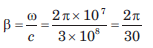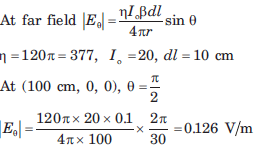QUESTION: 2

### A 25 A source operating at 300 MHz feeds a Hertizian dipole of length 4 mm situated at the origin. Consider the point P(10, 30o,90o). Que: The H at point P is

Solution: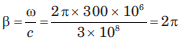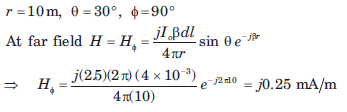QUESTION: 3

### A 25 A source operating at 300 MHz feeds a Hertizian dipole of length 4 mm situated at the origin. Consider the point P(10, 30o,90o). Que: The E at point P is

Solution: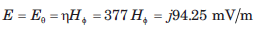QUESTION: 4

An antenna can be modeled as an electric dipole of length 4 m at 3 MHz. If current is uniform over its length, then radiation resistance of the antenna is

Solution: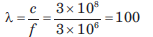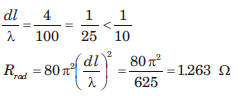QUESTION: 5

A antenna located on the surface of a flat earth transmit an average power of 150 kW. Assume that all the power is radiated uniformly over the surface of hemisphere with the antenna at the center. The time-average poynting vector at 50 km is

Solution: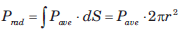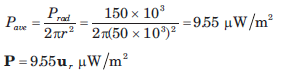QUESTION: 6

An L-band pulse radar has common transmitting and receiving antenna. The antenna having directive gain of 36 dB operates at 1.5 GHz and transmits 200 kW. The object is 120 km from the radar and its scattering cross section is 8 m2

Que: The magnitude of the incident electric field intensity of the object is

Solution:

Gd = 36 dB = 3981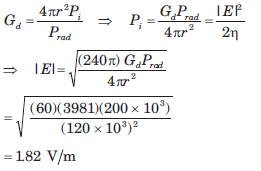QUESTION: 7

An L-band pulse radar has common transmitting and receiving antenna. The antenna having directive gain of 36 dB operates at 1.5 GHz and transmits 200 kW. The object is 120 km from the radar and its scattering cross section is 8 m2

Que: The magnitude of the scattered electric field at the radar is

Solution: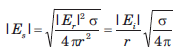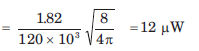QUESTION: 8

A transmitting antenna with a 300 MHz carrier frequency produces 2 kW of power. If both antennas has unity power gain, the power received by another antenna at a distance of 1 km is

Solution: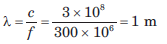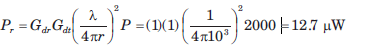QUESTION: 9

A bistatic radar system shown in fig. has following parameters: f = 5 GHz, Gdt = 34 dB, Gdr = 22 dB. To obtain a return power of 8 pW the minimum necessary radiated power is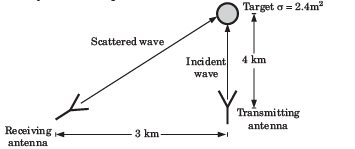Solution: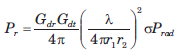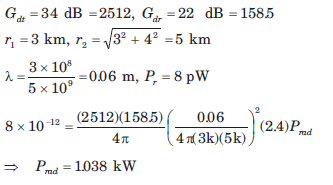QUESTION: 10

The radiation resistance of an antenna is 63Ω and loss resistance 7Ω If antenna has power gain of 16, then directivity is

Solution: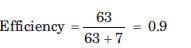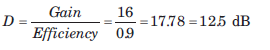QUESTION: 11

An antenna is desired to operate on a frequency of 40 MHz whose quality factor is 50. The bandwidth of antenna is

Solution: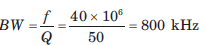QUESTION: 12

A thin dipole antenna is  λ/15 long. If its loss resistance is 1.2 Ω, the efficiency is

Solution: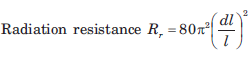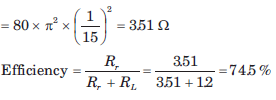QUESTION: 13

An array comprises of two dipoles that are separated by the wavelength. The dipoles are fed by currents of the same magnitude and phase.

Que: The array factor is

Solution: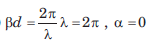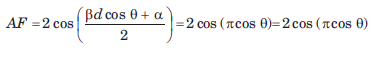QUESTION: 14

An array comprises of two dipoles that are separated by the wavelength. The dipoles are fed by currents of the same magnitude and phase.

Que: The nulls of the pattern occur when θ is

Solution:

cos (πcosθ) = 0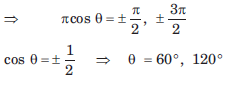QUESTION: 15

An array comprises of two dipoles that are separated by the wavelength. The dipoles are fed by currents of the same magnitude and phase.

Que: The maximum of the pattern occur at

Solution: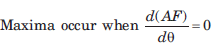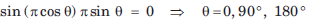Use Code STAYHOME200 and get INR 200 additional OFF Use Coupon Code